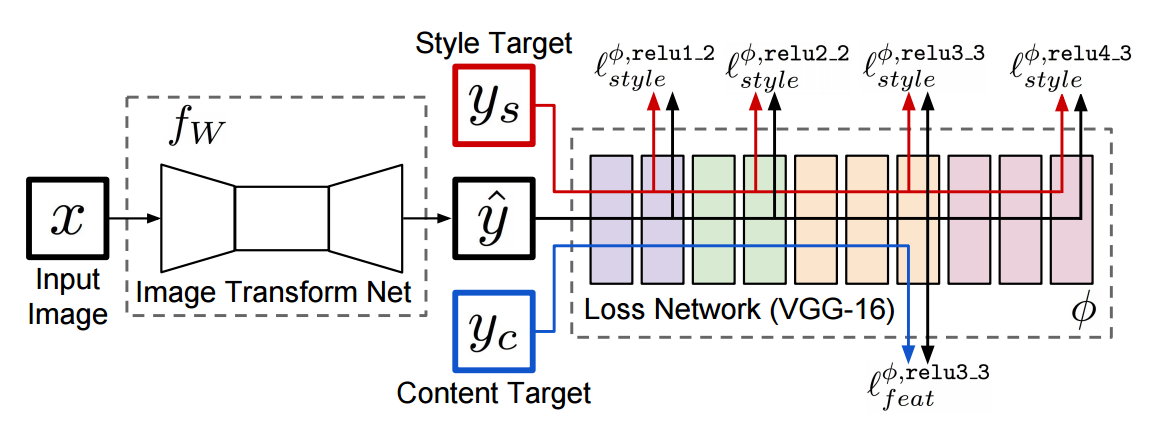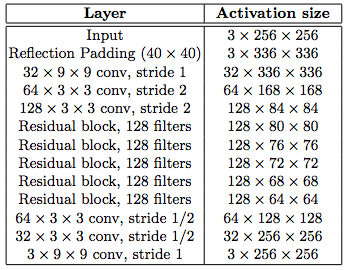# Fast (Feedforward) Style Transfer

Perceptual Losses for Real-Time Style Transfer and Super-Resolution is the second paper in my reading series for Neural Style Transfer, where Johnson et al. built on the work of Gatys et al. and used feedforward networks to stylize image order of magnitudes faster than the previous optimization approach.

### Intro

Two common approach for image transformation, where an input image is transformed into an output image:

• feed-forward CNN using per-pixel loss between the output and ground-truth image
• generate image by defining and optimizing perceptual loss based on high-level features extracted from pretrained networks

The paper combined the benefits of both approaches and proposed a system that

• train feed-forward network using use perceptual loss functions that depend on high-level features from a pretrained loss network
• allow the feed-forward network to learn a artistic style during training and can stylize images in real-time at test time

### MethodThe system consists of two components:

• image transformation network $f_W$: a deep residual CNN parameterized by $W$ and transforms input images $x$ into output images $\hat{y} = f_W(x)$
• loss network $\phi$: used to define several loss functions $l_1, \cdots, l_k$. Each loss function computes a scalar loss value $l_i(\hat{y}, y_i)$ that measures the difference between the output image $\hat{y}$ and a target image $y_i$.

The network is trained using SGD to minimize a loss functions of $$W^{\ast} = \text{argmin}_W \ \textbf{E}_{x, \{y_i\} } \left[ \sum_{i} \lambda_i l_i (f_W(x), y_i)\right]$$

• Inspired from the optimziation approach from Gatys et al. and others, the author use a pretrained network $\phi$ as a fixed loss network to define the loss functions.
• The loss network $\phi$ is used to define a feature reconstruction loss $l_{feat}^{\phi}$ and style reconstruction loss $l_{style}^{\phi}$ that measure differences in content and style between images.
• For each input image $x$, there is a content target $y_c$ and a style target $y_s$
• For style transfer, the content target $y_c$ is the input image $x$ and the style target $y_s$ is the designated style image. One network is trained for each style target.

### Image Transformation Network

The image transformation network is a Deep Residual Network. More detail can be found in the supplementary notes of the paper.### Loss Network

Johnson et al. defined the feature reconstruction loss and the style reconstruction loss in the same way as Gatys et al., though with a different notation. Let $\phi_{j}(x)$ be the $j$-th layer activations of the loss network for image $x$ with a shape $C_j \times H_j \times W_j$.

#### Feature Reconstruction Loss

• Euclidean distance between feautre representations $$l_{feat}^{\phi, j}(\hat{y},y) = \frac{1}{C_j H_j W_j} ||\phi_j(\hat{y}) - \phi_j(y)||^2_2$$
• Minimizing $l_{feat}^{\phi}$ would reserve the image content and overall spatial structure, but not the color, texture or exacte shape

#### Style Reconstruction Loss

• Squared Frobenius norm of the distance between the Gram matrices between the output and target image
• Gram matrix $G_j^\phi(x)$ is a $C_j \times C_j$ whose elements are the inner product between the two channels $c$ and $c'$ of activations $$G_j^\phi(x)_{c,c'} = \frac{1}{C_j H_j W_j} \sum_{h=1}^{H_j} \sum_{w=1}^{W_j} \phi_j(x)_{h,w,c} \phi_j(x)_{h,w,c'}$$ $$l_{style}^{\phi, j}(\hat{y},y) = ||G^\phi_j(\hat{y}) - G^\phi_j(y)||^2_F$$
• Minimizing $l_{style}^{\phi}$ would reserve the stylistic features, but not its spatial structure

#### Total Variation Regularization

Other than the $l_{feat}^{\phi}$ and $l_{style}^{\phi}$, Johnson et al. also defined a loss function $l_{TV}(\hat{y})$ to encourage spatial smoothness in the output image $\hat{y}$.

### Experiments and Training Details

• The goal of style transfer is to generate an image $\hat{y}$ that combines the content of the content target $y_c$ with the style of the style target $y_s$.
• Gatsy et al. formulate the problem as a optimization problem. An image $\hat{y}$ is generated by solving the problem $$\hat{y} = \text{argmin}_y \ \lambda_c l_{feat}^{\phi, j}(y, y_c) + \lambda_s l_{style}^{\phi, j}(y, y_s) + \lambda_{TV} l_{TV}(y)$$
• However, the method is slow since satifactory results takes about 500 iterations and each iterations requires a forward and a backward pass
• The result from the style transfer network is qualitatively similar to Gatsy et al’s method, but can be run in real time during test time
• Even though the style transfer network is trained on $256 \times 256$ images, they also perform satisfactorily in higher resolution of $512 \times 512$ and $1024 \times 1024$, achieving a performance comparable to 50-100 iterations of Gatsy et al’s method